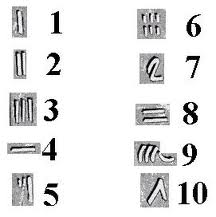Find all numbers which are equal to the sum of the factorial of their digits

0
86
• 1
•
•
•
•
•
•
•
•
•
1
ShareHope you have enjoyed https://www.techtravelhub.com/2011/12/factorial-how-i-coded-it.html
Now let me find out some number in such a way that sum of factorial of each digit of that number is equal to that number.
like-145 is a curious number, as 1! + 4! + 5! = 1 + 24 + 120 = 145
So the logic is —
1.Get the number
2. Find out each digit of that number
3. Find out factorial of that number
4. Sum of the factorials
5. Check if the original input is equal to the sum
6. if they matches print the result.

How to code:

2. Now I need to create a logic that will give me the digits from a number.

private void getDigit(int number)
{

int tmp = 1;
while(number > 0)
{
tmp = number % 10;
number /= 10;
System.out.println(tmp );
}
}

So now we have the numbers of each digit.

4. Sum of the factorials

so after getting factorials for each digit we need to sum these.let me write a function for it which will call factorial method and sum them up.

private int addfactOfDigits(int number) {
long result = 0;
int tmp = 1;
while(number > 0) {
tmp = number % 10;
//recursive factorial method more details on here
result += recfact_withoutstatic(tmp);
number /= 10;
}
return (int)result;
}
}

5. Check if the original input is equal to the sum

if (i== addfactOfDigits (i))
//i is the number we are passing to our function addfactOfDigits
System.out.println(i);
Don't miss out!

Receive top technical news, lesson ideas, travel tips and more!

Give it a try. You can unsubscribe at any time.